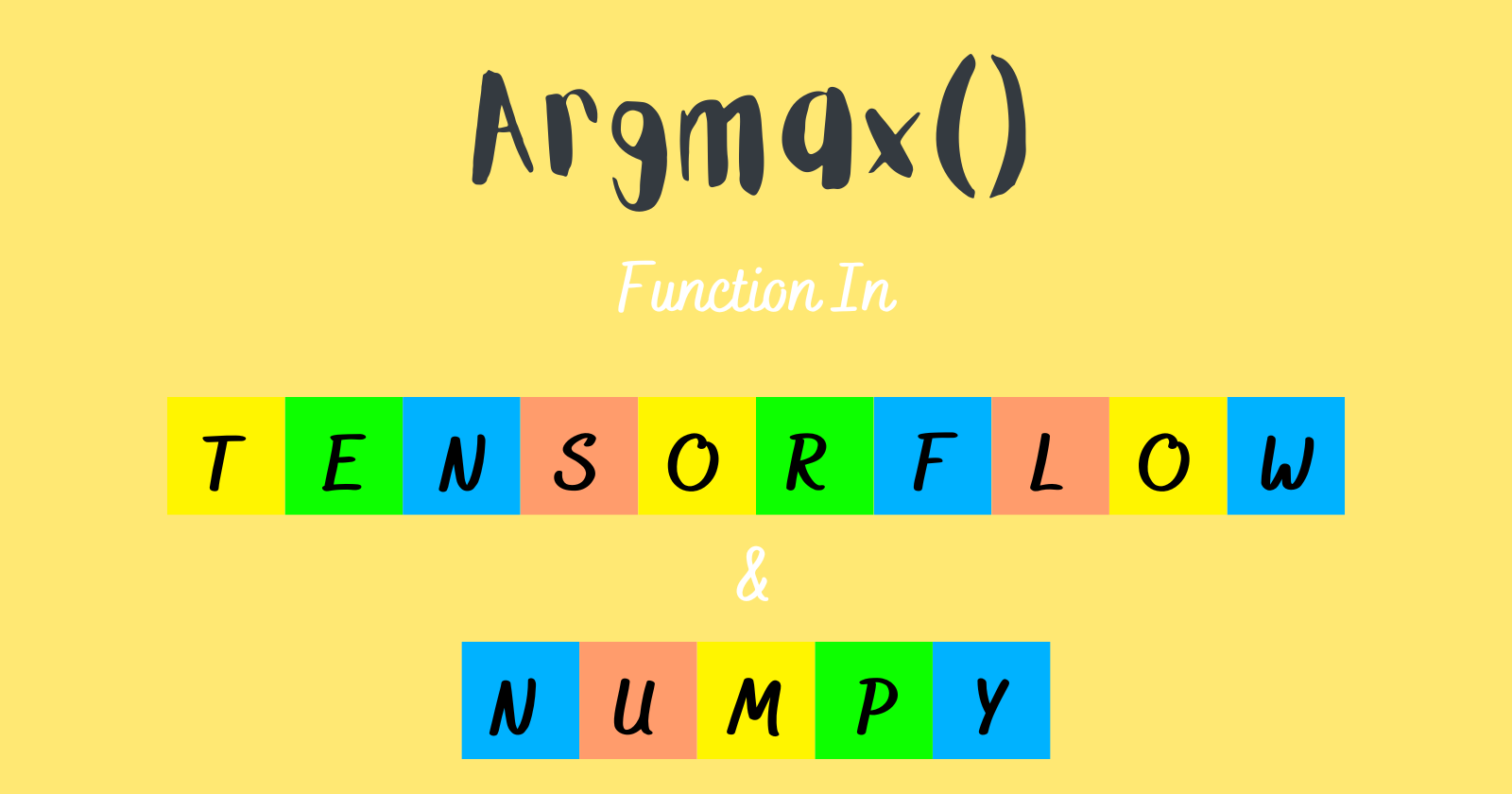## argmax() Function in NumPy and TensorFlow – Are They Same

Numpy is a Python library used for working with arrays. It provides multi-dimensional array objects, masked arrays, and matrices. To work with these arrays, NumPy provides some methods to carry out operations like linear algebra, statistical operations, shape manipulation, mathematical and logical operations, and much more.

The array object in NumPy is called `ndarray`, and dimensions are called `axes`.

Whereas TensorFlow is an open-source library developed by Google primarily to build deep learning and machine learning neural networks.

However, TensorFlow has some modules and methods to handle arrays or tensors in TensorFlow’s language.

In this article, we will see the `argmax()` function in both NumPy and TensorFlow, and ultimately we’ll discover if they provide the same functionality to handle arrays.

## Definition

First, we’ll decipher the definition of the `argmax()` function provided in the docs of both the libraries NumPy and TensorFlow.

### numpy.argmax

NumPy provides a function called argmax() that returns the indices of the maximum element of the array along an axis.

`numpy.argmax()` can be used to get the indices of the maximum values from the 1-dimensional and multi-dimensional arrays.

### tensorflow.math.argmax or tensorflow.argmax

TensorFlow has a module named `math` that provides the `argmax()` function, or we can call the argmax() function directly from the TensorFlow is also used to return the index of the largest value across the axes of the tensor or array.

## Syntax

Let’s look at the syntax since they both are used for similar actions.

### numpy.argmax

`numpy.argmax(a, axis=None, out=None)`

Parameters:

• `a` – The array you will work on
• `axis` – It is optional. We can specify an axis like 0 or 1 to find the maximum value index horizontally or vertically.
• `out` – By default, it is None. It provides a feature to insert the output to the out area, but the array should be of appropriate shape and dtype.

Return value

An array is returned with the indices of max values with the same shape as `a.shape` with the dimension along the axis removed.

### tensorflow.argmax

`tensorflow.argmax( input, axis=None, output_type=tf.dtypes.int64, name=None )`

Parameters:

• `input` – It is a tensor from which we’ll get the index of the highest values.
• `axis` – It is for specifying the axes to reduce the tensor. In the case of vector, we always use `axis=0`.
• `output_type` – It defines the dtype in which the returned result should be. The default value is int64.
• `name` – By default it is None. It is used to specify a name for the operation.

Return value

A tensor is returned with the indices of the highest values along the axes with the type of output_type.

## Code Example

Let’s see some examples.

### numpy.argmax

Output

Since we didn’t specify the axis argument in the argmax(), the array is flattened or considered a 1D array, so we got the index of the highest value from the input array.

Finding indices of the highest values along the axis

Output

Get a detailed explanation of numpy.argmax() function.

### tensorflow.argmax

Output

Unlike NumPy, TensorFlow provides additional information like the shape and dtype of the output.

Finding the indices of the highest values in a tensor along the specified axis

Output

## Conclusion

We already discussed that NumPy is primarily for numerical computation, or we often use it for handling complex array operations. In contrast, TensorFlow is used to build machine learning and deep learning neural networks for developing AI-based applications.

Both libraries have a `argmax()` function, and by this article, we meant to look at the function.

After seeing the definition, syntax, and code, we can conclude that there was a slight difference in syntax, but the function’s fundamentals were the same in both libraries.

Another minor difference was that TensorFlow provides additional info like the output tensor’s shape and dtype.

Now we got a pretty good idea about the argmax() function provided by both libraries.

That’s all for now

Keep Coding✌✌